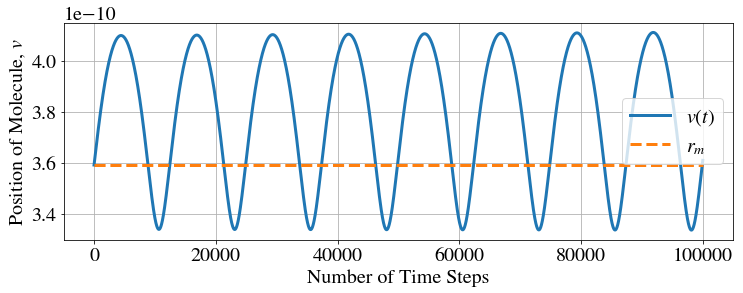# Lennard-Jones Potential¶

### Example - Chemistry¶

Last edited: March 22nd 2018

### Introduction to the Lennard-Jones Potential¶

The Lennard-Jones potential (also referred to as the L-J potential or 12-6 potential) is a simple physical model that approximates the interaction between a pair of neutral atoms or molecules.

The most common expressions for the L-J potential are:

\begin{equation} V_\mathrm{LJ} = 4\epsilon \left[\left(\frac{\sigma}{r}\right)^{12} - \left(\frac{\sigma}{r}\right)^6\right]=\epsilon \left[\left(\frac{r_m}{r}\right)^{12} - 2\left(\frac{r_m}{r}\right)^6\right], \label{potential} \end{equation}

where r is the distance between the particles. Here, $\epsilon$ is the depth (i.e. the minimum) of the potential well, reached at $r = r_m$, and $\sigma$ is the distance at which the inter-particle potential is zero. It can be shown that $r_m = 2^{1/6}\sigma$.

In :
%matplotlib inline
import numpy as np
import matplotlib.pyplot as plt
from matplotlib import rc

In :
newparams = {'figure.figsize': (12, 4), 'axes.grid': False,
'lines.markersize': 6, 'lines.linewidth': 3,
'font.size': 20, 'mathtext.fontset': 'stix',
'font.family': 'STIXGeneral'}
plt.rcParams.update(newparams)


After importing the necessary packages, we obtain the following graph for $\epsilon = \sigma = 1$:

In :
def plotV(r, eps, sig, xlim, ylim):
""" Function for plotting the Lennard-Jones potantial with given parameter values. """

V_LJ = 4*eps*((sig/r)**12-(sig/r)**6)

plt.plot(r, V_LJ)
plt.ylim(ylim)
plt.xlim(xlim)
plt.ylabel(r"$V_{LJ}(r)$")
plt.xlabel(r"$r$")

xlim = [0.8, 2]
ylim = [-1.5, 2]
r = np.linspace(0.5, 2, 100)
eps = 1
sig = 1
plotV(r, eps, sig, xlim, ylim)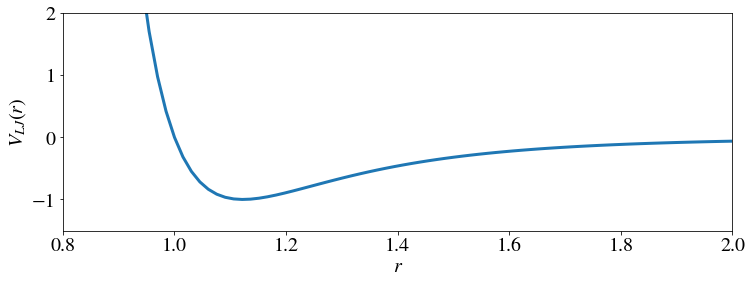As we can see, we find a global minimum and expect oscillations about this equilibrium as long as the total energy, $E_\mathrm{total} = E_\mathrm{kin} + E_\mathrm{pot}$ , does not exceed zero.

### Parameters for our Particular Example¶

In this example, we will consider the Lennard-Jones Potential for two H2O molecules. The parameters are:

• $\sigma=0.326 \times 10^{-9}$ m
• $\epsilon=1.08\times 10^{-21}$ J

Note that the orientation-dependent interaction between the water dipoles is not included here explicitly. We obtain the following plot:

In :
r = np.linspace(3e-10, 7e-10, 100)
eps = 1.08e-21
sig = 0.32e-9
ylim = [-1.5e-21, 1.5e-21]
xlim = [2.5e-10, 7e-10]

plotV(r, eps, sig, xlim, ylim)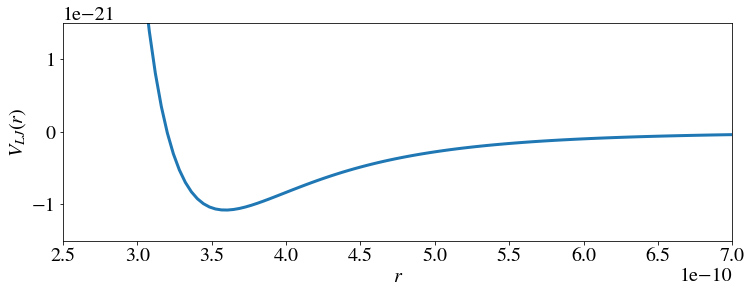### The Problem¶

We will now analyze oscillations about the equilibrium in a classical manner.

• First, we want to examine the frequency of small oscillations near $r = r_m$.
• To do this, we approximate the force near $r = r_m$.
• Thereafter, we want to compute the period of oscillations for larger perturbations when the linearization is a poor approximation.

#### Deriving the Force from the Potential¶

Let us calculate the force from the potential by taking the derivative of the potential:

\begin{equation} F = -\frac{\mathrm{d}V}{\mathrm{d}r} = \frac{12\epsilon}{r_m}\left[\left(\frac{r_m}{r}\right)^{13} - \left(\frac{r_m}{r}\right)^7\right]. \label{force} \end{equation}

Note that $F(r_m)=0$, hence $r=r_m$ is an equilibrium point. Now we Taylor expand the force around this point.

We write $$F(r_m + \Delta r) = F(r_m) + F'(r_m)\Delta r + \mathcal{O}(\Delta r^2) = 0 + F'(r_m)\Delta r + \mathcal{O}(\Delta r^2).$$

Now, we need to determine $F'(r)=\frac{\mathrm{d}F}{\mathrm{d}r}$:

\begin{equation} F'(r) = \frac{12\epsilon}{r_m^2}\left[-13\left(\frac{r_m}{r}\right)^{14} + 7\left(\frac{r_m}{r}\right)^8\right]. \label{forcederivative} \end{equation}

Substituting $r=r_m$ yields $$F'(r_m) = -\frac{72\epsilon}{r_m^2}.$$ Using this result, we find the force near $r_m$, $$F(r_m + \Delta r) \approx -\frac{72\epsilon}{r_m^2}\Delta r.$$

#### The Frequency $f$ of Small Oscillations¶

We can now compute the frequency of small oscillations about the equilibrium by writing $$m\frac{\mathrm{d^2}\Delta r}{\mathrm{d}t^2}= F(r_m + \Delta r) \approx -\frac{72\epsilon}{r_m^2}\Delta r,$$ where $m$ is the effective mass of the water-water molecule system. This describes harmonic oscillations with a frequency $$\omega^2 = (2\pi f)^2 = \frac{72\epsilon}{mr_m^2}$$ We use the values for $\epsilon$ and $r_m = 2^{1/6}\sigma$ from above, and $m\approx 9m_\mathrm{proton}$ to calculate the frequency:

In :
m = 9*1.67e-27
r_m = 2**(1/6)*sig

f = np.sqrt(72*eps/(m*r_m**2))/(2*np.pi)
print("f = %.4e" % (f))

f = 1.0079e+12


The result is $$f = \frac{\omega}{2\pi} = \frac{1}{2\pi}\sqrt{\frac{72\epsilon}{mr_m^2}}=1.01 \times 10^{12}\,\mathrm{Hz}.$$

### Expanding the Problem¶

So far, we have examined the frequency near $r = r_m$ where the dynamics can be approximated by that of a harmonic oscillator. This was simple and we did not need any computational tools. 􏰎However, what happens if we look at oscillations further away from $r = r_m$? 􏰎If we look at our plot of the L-J potential, we can see that the graph is not symmetric about the equilibrium. During oscillations, we would expect the molecules to spend more time to the right of the equilibrium $(r > r_m)$ then to the left of it. Let us study this phenomenon numerically.

#### Nonlinear Oscillations¶

Now, we will solve the exact dynamic equation $$m\ddot{r}=F(r),$$ where the dots indicate time derivatives.

We transform this ODE into a set of first-order ODEs and employ Euler’s method to solve it. We do this by introducing new variables $v$ and $w$, with $v = r$ and $w = \dot{r}$. Substitution into the equation \eqref{force} gives $$\dot{w} = \ddot{r} = \frac{F(r)}{m} = \frac{12\epsilon}{mr_m}\left[\left(\frac{r_m}{v}\right)^{13} - \left(\frac{r_m}{v}\right)^7\right]$$

#### Euler's Method: Set of Equations¶

This gives the following set of equations

\begin{align} \dot{v} &= w,\\ \dot{w} &= \frac{12\epsilon}{mr_m}\left[\left(\frac{r_m}{v}\right)^{13} - \left(\frac{r_m}{v}\right)^7\right]. \end{align}

For our initial conditions, we choose $v(0) = r_m$ and $w(0)>0$. Following the notation from the module Euler's Method, we can now write the Euler method as (with $v_1 = v(0), w_1 = w(0)$)

\begin{align} v_{n+1} &= v_n + h\cdot w_n,\\ w_{n+1} &= w_n + h\cdot \frac{12\epsilon}{mr_m}\left[\left(\frac{r_m}{v_n}\right)^{13} - \left(\frac{r_m}{v_n}\right)^7\right], \end{align}

where $h$ is the time step.

This can be implemented as follows:

In :
def euler_oscil(w0, h, n_tot):
""" A function which uses euler's method to calculate oscillations and then plots it.

Arguments:
w           initial value of w
h           time step
n_tot       total number of time steps
"""
v = np.zeros(n_tot)
w = np.zeros(n_tot)

v = r_m
w = w0

for n in range(n_tot-1):
v[n+1] = v[n] + h*w[n]
w[n+1] = w[n] + h*12*eps/(r_m*m)* ( (r_m/v[n])**13 - (r_m/v[n])**7 )

plt.plot(v)
plt.plot([0, n_tot], [r_m, r_m],'--')
plt.legend([r"$v(t)$", "$r_m$"])
plt.ylabel(r"Position of Molecule, $v$")
plt.xlabel(r"Number of Time Steps")
plt.grid();


#### Oscillations near $r=r_m$¶

We choose step size $h=10^{-15}\,\mathrm{s}$, and set $\frac{1}{2}m\omega^2 = 0.001\epsilon$. This corresponds to very small oscillations because the kinetic energy is much smaller than $\epsilon$ and, therefore, we are deep down in the potential well where the oscillations are harmonic. We plot the vector $v$ which represents the position of the molecule vs. time steps.

In :
w0 = np.sqrt(2*0.001*eps/m)  # Initial value for w
h = 1e-15  # Time step
n_tot = 1100  # Total number of time steps

euler_oscil(w0, h, n_tot)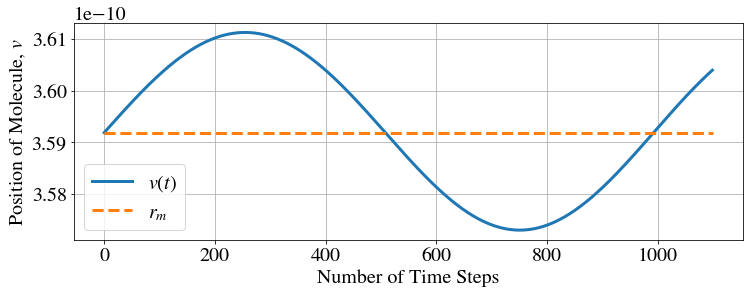We find the following features:

• The oscillations around the equilibrium $r = r_m$ seem harmonic.

• One period seems to be approximately 1000 time steps, each of length $10^{-15}$, i.e. we have $T\approx 1000\cdot 10^{-15} = 10^{-12}$, in good agreement with the result from the linear analysis.

#### Oscillations further up the Potential Well¶

Now let’s see what the oscillations look like when we add kinetic energy. We set $\frac{1}{2}m\omega^2 = 0.3\epsilon$ and $n_\mathrm{tot} = 1270$. We have to increase the number of time steps $n_\mathrm{tot}$ because we expect the period to be longer.

In :
w0 = np.sqrt(2*0.3*eps/m)  # Initial value for w
h = 1e-15  # Time step
n_tot = 1270  # Total number of time steps

euler_oscil(w0, h, n_tot)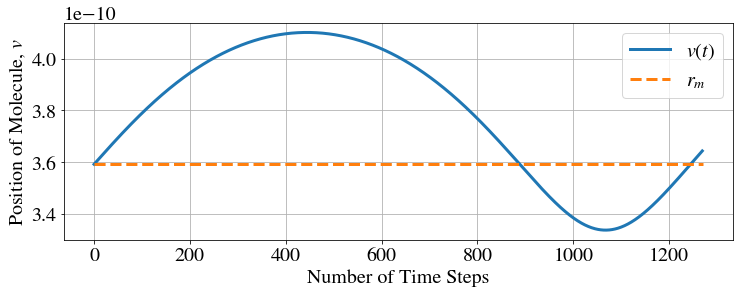• We can clearly see how the molecule spends more time on the right side $(r > r_m)$ of the potential well which we expected from the L-J plot.
• In this case, the period is about $T=1270\cdot10^{−15}$ s. Since $T$ increases with the amplitude, we no longer have harmonic oscillations.
• Note that we treat this microscopic system classically and not quantum mechanically. However, it gives us some ideas about how a full quantum mechanical treatment would differ from that of a quantum mechanical harmonic oscillator.

#### Repeating Oscillations¶

So far, we have only looked at oscillations for one period. If we increase the number of oscillations, we would expect neither diminishing nor increasing amplitudes since energy is conserved. Unfortunately, Euler’s method contains errors which can be large enough to clearly violate energy conservation in our solution. Hence, the amplitude may not be constant.

In :
w0 = np.sqrt(2*0.3*eps/m)  # Initial value for w
h = 1e-15  # Time step
n_tot = 10000  # Total number of time steps

euler_oscil(w0, h, n_tot)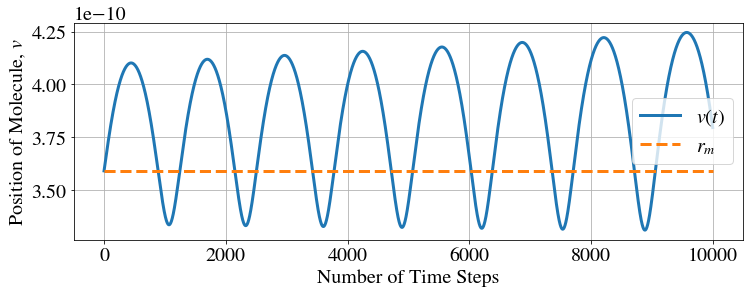As the number of oscillations increases, the molecules move further away from equilibrium with each oscillation which would correspond to an increase in energy.

### Error in the Euler Method¶

Remember that this method is an approximation of the solution. Each time we calculate a new step, we make an error. This error results in an increase in the amplitude of each new oscillation. The error can be reduced by using better but more complicated approximations, or by reducing the step size $h$.

In the next plot,we have reduced the step size to $h = 10^{−16}$ s. We have to increase the number of calculations to $n_\mathrm{tot} = 100 000$ so as to obtain the same amount of oscillations. This demands more computational time but reduces the error.

In :
w0 = np.sqrt(2*0.3*eps/m)  # Initial value for w
h = 1e-16  # Time step
n_tot = 100000  # Total number of time steps

euler_oscil(w0, h, n_tot)